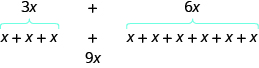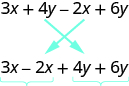## Simplifying Algebraic Expressions

### Learning Outcomes

• Identify the coefficient of a variable term
• Recognize and combine like terms in an expression
• Use the order of operations to simplify expressions containing like terms

## Identify Terms, Coefficients, and Like Terms

In mathematics, we may see expressions such as $x+5,\dfrac{4}{3}{r}^{3}$, or $5m-2n+6mn$. Algebraic expressions are made up of terms. A term is a constant or the product of a constant and one or more variables. Some examples of terms are $7,y,5{x}^{2},9a,\text{ and }13xy$.

In the expression $x+5$, $5$ is called a constant because it does not vary and $x$ is called a variable because it does. (In naming the variable, ignore any exponents or radicals containing the variable.) An algebraic expression is a collection of constants and variables joined together by the algebraic operations of addition, subtraction, multiplication, and division.

The constant that multiplies the variable(s) in a term is called the coefficient. We can think of the coefficient as the number in front of the variable. The coefficient of the term $3x$ is $3$. When we write $x$, the coefficient is $1$, since $x=1\cdot x$. The table below gives the coefficients for each of the terms in the left column.

Term Coefficient
$7$ $7$
$9a$ $9$
$y$ $1$
$5{x}^{2}$ $5$

An algebraic expression may consist of one or more terms added or subtracted. The table below gives some examples of algebraic expressions with various numbers of terms. Notice that we include the operation before a term with it. Think of the operation as belonging to the term it precedes.a

Expression Terms
$7$ $7$
$y$ $y$
$x+7$ $x,7$
$2x+7y+4$ $2x,7y,4$
$3{x}^{2}+4{x}^{2}+5y+3$ $3{x}^{2},4{x}^{2},5y,3$

### example

Identify each term in the expression $9b+15{x}^{2}+a+6$. Then identify the coefficient of each term.

Solution:
The expression has four terms. They are $9b,15{x}^{2},a$, and $6$.

• The coefficient of $9b$ is $9$.
• The coefficient of $15{x}^{2}$ is $15$.
• Remember that if no number is written before a variable, the coefficient is $1$. So the coefficient of $a$ is $1$.
• The coefficient of a constant is the constant, so the coefficient of $6$ is $6$.

### try it

Some terms share common traits. Look at the following terms. Which ones seem to have traits in common?

$5x,7,{n}^{2},4,3x,9{n}^{2}$
Which of these terms are like terms?

• The terms $7$ and $4$ are both constant terms.
• The terms $5x$ and $3x$ are both terms with $x$.
• The terms ${n}^{2}$ and $9{n}^{2}$ both have ${n}^{2}$.

Terms are called like terms if they have the same variables and exponents. All constant terms are also like terms. So among the terms $5x,7,{n}^{2},4,3x,9{n}^{2}$,

• $7$ and $4$ are like terms.
• $5x$ and $3x$ are like terms.
• ${n}^{2}$ and $9{n}^{2}$ are like terms.

### Like Terms

Terms that are either constants or have the same variables with the same exponents are like terms.

Like terms are terms where the variables match exactly (exponents included). Examples of like terms would be $5xy$ and $-3xy$, or $8a^2b$ and $a^2b$, or $-3$ and $8$.

### example

Identify the like terms:

1. ${y}^{3},7{x}^{2},14,23,4{y}^{3},9x,5{x}^{2}$
2. $4{x}^{2}+2x+5{x}^{2}+6x+40x+8xy$

## Simplify Expressions by Combining Like Terms

We can simplify an expression by combining the like terms. What do you think $3x+6x$ would simplify to? If you thought $9x$, you would be right!

We can see why this works by writing both terms as addition problems.Add the coefficients and keep the same variable. It doesn’t matter what $x$ is. If you have $3$ of something and add $6$ more of the same thing, the result is $9$ of them. For example, $3$ oranges plus $6$ oranges is $9$ oranges. We will discuss the mathematical properties behind this later.

The expression $3x+6x$ has only two terms. When an expression contains more terms, it may be helpful to rearrange the terms so that like terms are together. The Commutative Property of Addition says that we can change the order of addends without changing the sum. So we could rearrange the following expression before combining like terms.Now it is easier to see the like terms to be combined.

### Combine like terms

1. Identify like terms.
2. Rearrange the expression so like terms are together.
3. Add the coefficients of the like terms.

If we have like terms, we are allowed to add (or subtract) the numbers in front of the variables, then keep the variables the same. Kind of like saying four pens plus three pens equals seven pens. But two pens plus six pencils can’t be combined and simplified because they are not “like terms”. As we combine like terms we need to interpret subtraction signs as part of the following term. This means if we see a subtraction sign, we treat the following term like a negative term. The sign always stays with the term.

This is shown in the following examples:

### example

Simplify the expression: $3x+7+4x+5$.

### Example

Combine like terms:  $5x-2y-8x+7y$

### Example

Combine like terms:  $x^2-3x+9-5x^2+3x-1$

In the video that follows, you will be shown another example of combining like terms.  Pay attention to why you are not able to combine all three terms in the example.

### example

Simplify the expression: $8x+7{x}^{2}+{x}^{2}+4x$.

### try it

In the following video, we present more examples of how to combine like terms given an algebraic expression.

## Contribute!

Did you have an idea for improving this content? We’d love your input.